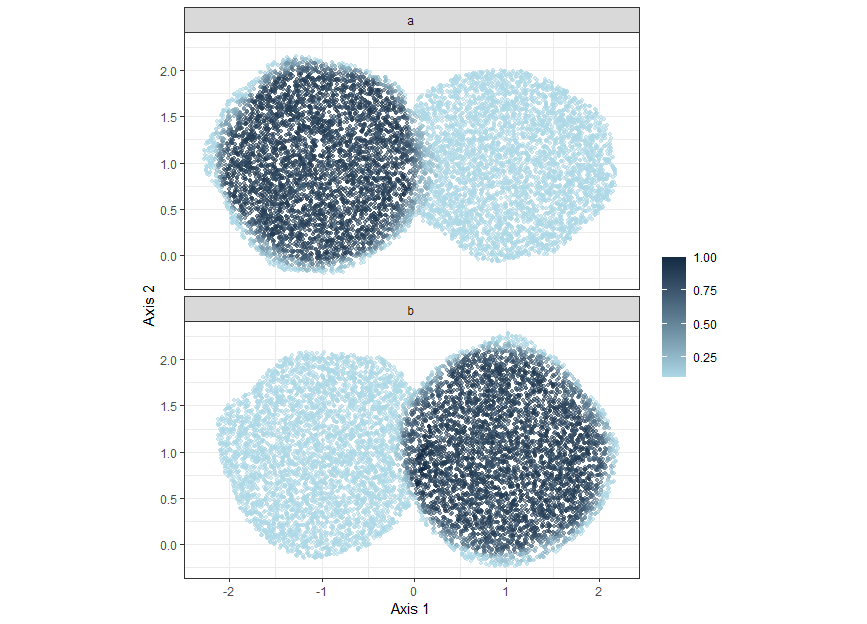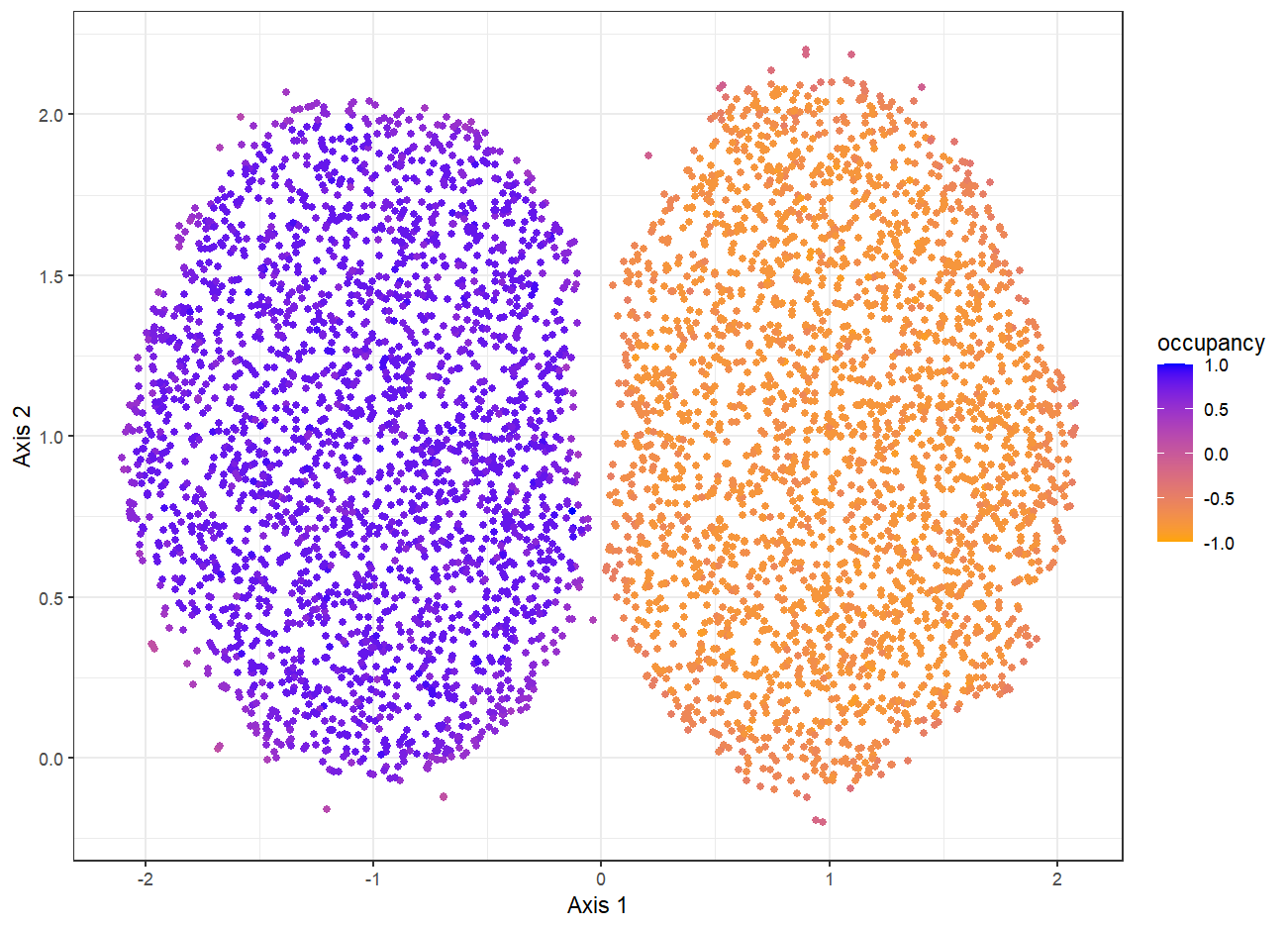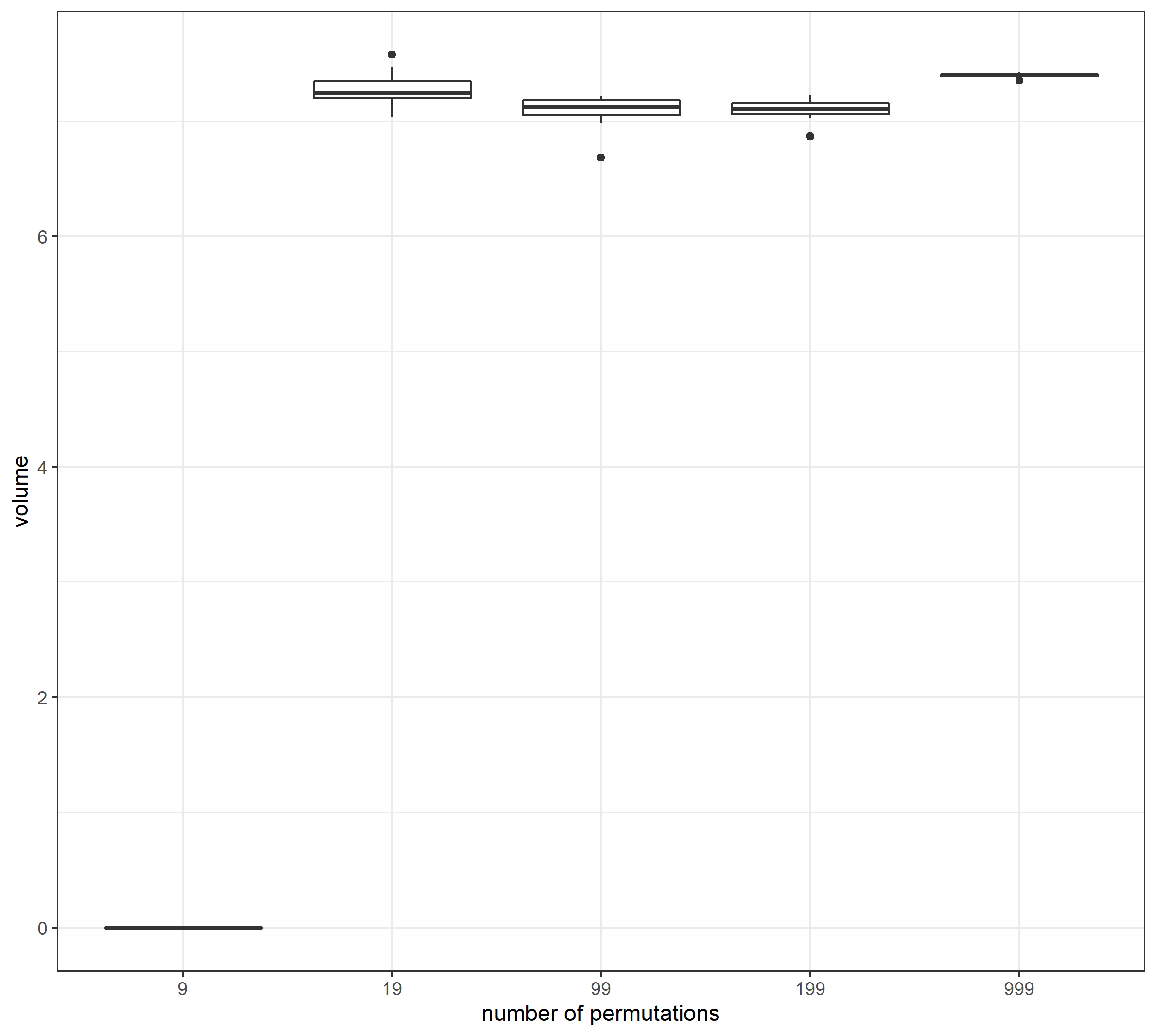# Introduction to occupancy

The occupancy routine provides a framework to join and to calculate summary statistics for multiple hypervolumes simultaneously by leveraging on the concept of occupancy, a function of the number of hypervolumes including a point in the trait space. At first, a set of random points covering all the hypervolumes is selected through one of the two methods available. The subsampling method joins the random points of the input hypervolumes and then selects a uniformly distributed subset of them. The method box creates a bounding box around the union of the q hypervolumes that is then filled with random points drawn from a uniform distribution at a specified density. Secondly, a function (e.g. mean, sum) is applied to each random point. Occupancy can be calculated for groups of observations such that emerging from repeated measures over space, time or treatments and is intended to reflect the heterogeneity of trait space utilization by multiple hypervolumes, that could be in turn associated with relevant ecological processes.
The occupancy routine comes with a permutation test to detect between-group differences in occupancy values. For each pairwise group comparison, the original hypervolumes are randomly assigned to one of the two groups under comparison. The test itself is performed by counting the number of times the observed differences are smaller or greater than those expected by chance, or by combining them for obtaining a two-tailed test.

## SIMULATED EXAMPLE

We generated hypervolumes by picking 100 points randomly within a circle of radius 1. The first group (a) is composed of one hypervolume centered at coordinates x = -1 and y = 1 and 9 hypervolumes at coordinates x = 1 and y = 1. The second group (b) is built using the same strategy but by inverting x and y the coordinates. We then estimated occupancy as the average number of hypervolumes including a given random point. We tested each scenario (number of hypervolumes) with an increasing number of permutations (9, 19, 99, 199, 999) for 19 times in order to evaluate the consistency of the results. Moreover, we performed a two-tailed test with a probability threshold of 0.05 and reported the volume resulting from the permutation test was used as the target metric. Given the simulation setting, we expect a volume of the significant fraction to be close to the true value of 2$$\pi$$=6.28, corresponding to both hypervolumes together. The uncertainty about the volume is due to the uncertainty during hypervolume construction.

library(tidyverse)
library(ggpubr)
library(hypervolume)

data(circles)

# set seed for reproducibility
set.seed(42)

# create gaussian hypervolumes from points using lapply
# and transform the resulting list in a HypervolumeList
hyper_list <- lapply(circles, function(x) hypervolume_gaussian(x))
hyper_list <- hypervolume_join(hyper_list)

# create labels to divide the hypervolumes in the resulting HypervolumeList
# into two groups
group_labels <- rep(letters[1:2], each = 10)

# create an occupancy object from the hyper_list
# we will use the box method, and the summary statistics of each random point
# calculated as the mean value
hyper_occupancy <- hypervolume_n_occupancy(hyper_list,
method = "box",
classification = group_labels,
box_density = 5000,
FUN = mean)

# transform the object hyper_occupancy to a data.frame for plotting
plot_hyper_occupancy <-  hypervolume_to_data_frame(hyper_occupancy)

# plot the hyper_occupancy, by removing ValueAtRandomPoints equal to 0
# and by taking a subsample for shortening the time needed to generate the plot
plot_hyper_occupancy %>%
select(X.1, X.2, Name, ValueAtRandomPoints) %>%
filter(ValueAtRandomPoints != 0) %>%
group_by(Name) %>%
sample_n(10000) %>%
ungroup() %>%
ggplot(aes(X.1, X.2, col = ValueAtRandomPoints), alpha = 0.7) +
geom_point(size = 1, alpha = 0.5) +
theme_bw() +
facet_wrap(~ Name, ncol = 1) +
labs(x = "Axis 1", y = "Axis 2", color = "") +
scale_colour_continuous(high = "#132B43", low = "#add8e6") +
coord_fixed()Figure 1. Occupancy of two groups of hypervolumes. The first group (a) has most of the hypervolmes on the left while the second group (b) has most of the hypervolumes on the right. Occupancy is calculated for each random points as the fraction of hypervolumes enclosing the given random point.

The permutation test can be performed using the functions hypervolume_n_occupancy_permute() and hypervolume_n_occupancy_test().

# set the folder to store the permutate hypervolumes
folder_name <- paste("circles_99", "20", sep = "_")

# permute the hypervolumes 99 times
hyper_op <- hypervolume_n_occupancy_permute(folder_name, hyper_occupancy,
hyper_list,
n = 99,
cores = 4)
# perform a two-tailed permutation test
hyper_op_test<- hypervolume_n_occupancy_test(hyper_occupancy,
hyper_op,
alternative = "two_sided")

# transform the results of the permutation test into a data.frame for plotting
# we will take a subset of the random points to reduce plotting times
plot_circles_test <- hypervolume_to_data_frame(hyper_occupancy_permute_test_ts) %>%
rename(occupancy = ValueAtRandomPoints) %>%
filter(occupancy != 0) %>%
sample_n(5000)

# plot the results with ggplot2
ggplot(plot_circles_test) +
geom_point(aes(x = X.1, y = X.2, col = occupancy)) +
theme_bw() +
scale_color_gradient(low = "orange", high = "blue", limits = c(-1, 1)) +
labs(x = "Axis 1", y = "Axis 2", color = "")

We can assess how many permutations we need to have stable results. We will explore different number of permutation multiple times (19). Results show that we need at least 19 permutations to detect a significant differences at 0.05 significance level.Figure 2. Results of a two-tailed permutation test perfomed on the circles dataset. The permutation test correctly identifies regions preferentially occupied by group a on the left and by group b on the right. Reported values represent the differences between occupancy values (calculated as the fraction of hypervolumes enclosing a given random point) of group a and those of group b. This is the reason why negative values are also reported.

# number of iterations
N <- 19

### 9 permutations
# initialize an empty vector to store the volume of the significant
# fraction evaluated with the permutation test
res_9 <- c()

for(i in 1:N){
# name of the folder to store the permutations
# 9 = number of permutation
# i = number of iteration
# 20 = number of hypervolumes in hyper_list
folder_name <- paste("vol_same_coord_9", i, "20", sep = "_")

# perform 9 hypervolume permutation
hyper_op <- hypervolume_n_occupancy_permute(folder_name, hyper_occupancy,
hyper_list,
n = 9,
cores = 4)

# perform a two-tailed test
hyper_op_test <- hypervolume_n_occupancy_test(hyper_occupancy,
hyper_op,
alternative = "two_sided")

# store the volume of the signifiant fraction
res_9 <- c(res_9, hyper_op_test@Volume)
}

### 19 permutations
res_19 <- c()

for(i in 1:N){
folder_name <- paste("vol_same_coord_19", i, "16", sep = "_")
hyper_occupancy_permute <- hypervolume_n_occupancy_permute(folder_name, hyper_occupancy,
hyper_list,
n = 19, cores = 4)
hyper_occupancy_permute_test_ts <- hypervolume_n_occupancy_test(hyper_occupancy,
hyper_occupancy_permute,
alternative = "two_sided")

res_19 <- c(res_19, hyper_occupancy_permute_test_ts@Volume)
}

### 99 permutations
res_99 <- c()

for(i in 1:N){
folder_name <- paste("vol_same_coord_99", i, "16", sep = "_")
hyper_occupancy_permute <- hypervolume_n_occupancy_permute(folder_name, hyper_occupancy,
hyper_list,
n = 99, cores = 4)
hyper_occupancy_permute_test_ts <- hypervolume_n_occupancy_test(hyper_occupancy,
hyper_occupancy_permute,
alternative = "two_sided")

res_99 <- c(res_99, hyper_occupancy_permute_test_ts@Volume)
}

### 199 permutations
res_199 <- c()

for(i in 1:N){
folder_name <- paste("vol_same_coord_199", i, "16", sep = "_")
hyper_occupancy_permute <- hypervolume_n_occupancy_permute(folder_name, hyper_occupancy,
hyper_list,
n = 199, cores = 4)

hyper_occupancy_permute_test_ts <- hypervolume_n_occupancy_test(hyper_occupancy,
hyper_occupancy_permute,
alternative = "two_sided")

res_199 <- c(res_199, hyper_occupancy_permute_test_ts@Volume)
}

res_199 <- na.omit(res_199)
range(res_199)
mean(res_199)
median(res_199)
quantile(res_199, c(0.025, 0.975))

### 999 permutations
res_999 <- c()

for(i in 1:N){
folder_name <- paste("vol_same_coord_999", i, "16", sep = "_")
hyper_occupancy_permute <- hypervolume_n_occupancy_permute(folder_name, hyper_occupancy,
hyper_list,
n = 999, cores = 4)

hyper_occupancy_permute_test_ts <- hypervolume_n_occupancy_test(hyper_occupancy,
hyper_occupancy_permute,
alternative = "two_sided")

res_999 <- c(res_999, hyper_occupancy_permute_test_ts@Volume)
}

res_999 <- na.omit(res_999)Figure 3. Number of permutations needed to have significant results for the circle dataset. A volume of 6.28 (the sum of the volume of both groups) is expected, because of the way the simulation was built. Group a have in fact significantly greater occupancy on the left while group by on the right (see figure 1). A minimum of 19 permutations are needed to have significant results at 0.05 level of significance.

## REAL CASE EXAMPLE

We assessed the climatic niche occupancy of two highly speciose plant genera, Acacia and Pinus, to evaluate patterns in climatic preferences within each genus. Here, occupancy represent the mean number of species that occupy a given point in the multidimensional space. For each species within these genera (60 and 63 for Acacia and Pinus, respectively) we downloaded occurrence data from the BIEN dataset (Maitner, 2022). Climatic data for each occurrence was obtained from worldclim (Fick and Hijmans, 2017) using the raster package (Hijmans et al. 2022). As in Blonder et al. (2018), we selected three climate variables: mean annual temperature, mean annual precipitation, and precipitation in warmest quarter/(precipitation in warmest quarter + precipitation in coldest quarter). For each species, niches were built using the function hypervolume_gaussian with standard settings. Climate layers were z- transformed (centered relative to mean and scaled relative to standard deviation) prior to hypervolume construction. Occupancy at genus level was obtained with the function hypervolume_n_occupancy using the box method and the mean as the summary statistics.

# set.seed
set.seed(42)

# get climatic variables using the raster package
climate <- raster::getData('worldclim', var='bio', res=10)

# Create a new climate variable
bio20<-climate[]/(climate[]+climate[])
slot(bio20@data, "names")<-"bio20"

# Restrict our stack to 3 variables with low correlation
climate<-raster::stack(climate[[c(1,12)]],bio20)
rm(bio20)

# Do a z-transform on our data
climate <- raster::scale(climate)

data("acacia_pinus")

# get unique species
species_unique <- unique(acacia_pinus\$species)

# create hypervolumes for each species with a loop
# at first, create a list to store the hypervolumes
# and also a vector to store the genus information
hyper_list <- list()
genus_unique <- c()

for(i in 1:length(species_unique)){
# get climatic data of the ith species
data_i <- acacia_pinus %>%
filter(species == species_unique[i]) %>%
select(longitude, latitude) %>%
raster::extract(x = climate,
y = .)

# remove NA data
data_i <- na.omit(data_i)

# calculate the hypervolume assigning the species name
hyper_list[[i]] <- hypervolume(data_i, name = species_unique[i], verbose = FALSE)
# store the genus level information
genus_unique <- c(genus_unique, unlist(strsplit(species_unique[i], " ")))
print(paste(species_unique[i], ": done", sep = ""))
}

# transform hyper_list in an HypervolumeList
hyper_list <- hypervolume_join(hyper_list)

# increase box_density if you want more accurate results
# with mean as summary statistics
hyper_occupancy <- hypervolume_n_occupancy(hyper_list,
classification = genus_unique,
method = "box",
FUN = "mean")

# transform hyper_occupancy into a data.frame for plotting
climatic_occupancy <- hypervolume_to_data_frame(hyper_occupancy) %>%
filter(ValueAtRandomPoints != 0) %>%
group_by(Name) %>%
sample_n(2000) %>%
ungroup() %>%
sample_n(nrow(.)) %>%
rename(genus = Name, occupancy = ValueAtRandomPoints)

# plot bio1 vs bio12
g1_12_points <- ggplot(climatic_occupancy) +
geom_point(aes(bio1, bio12, col = genus, size = occupancy), alpha = 0.1) +
theme_bw() +
scale_size_continuous(limits = c(0, 1)) +
scale_colour_manual(values = c("#FFC20A", "#0C7BDC")) +
labs(title = "bio1 - bio12")

# plot bio1 vs bio20
g1_20_points <- ggplot(climatic_occupancy) +
geom_point(aes(bio1, bio20, col = genus, size = occupancy), alpha = 0.1) +
theme_bw() +
scale_size_continuous(limits = c(0, 1)) +
scale_colour_manual(values = c("#FFC20A", "#0C7BDC")) +
labs(title = "bio1 - bio20")

# plot bio12 vs bio20
g12_20_points <- ggplot(climatic_occupancy) +
geom_point(aes(bio12, bio20, col = genus, size = occupancy), alpha = 0.1) +
theme_bw() +
scale_size_continuous(limits = c(0, 1)) +
scale_colour_manual(values = c("#FFC20A", "#0C7BDC")) +
labs(title = "bio12 - bio20")

# compose the plot
ggarrange(g1_12_points, g1_20_points, g12_20_points,
common.legend = TRUE,
legend = "right",
ncol = 3,
nrow = 1)